# Douglas Rachford Proximal Splitting¶

$\newcommand{\dotp}{\langle #1, #2 \rangle}$ $\newcommand{\enscond}{\lbrace #1, #2 \rbrace}$ $\newcommand{\pd}{ \frac{ \partial #1}{\partial #2} }$ $\newcommand{\umin}{\underset{#1}{\min}\;}$ $\newcommand{\umax}{\underset{#1}{\max}\;}$ $\newcommand{\umin}{\underset{#1}{\min}\;}$ $\newcommand{\uargmin}{\underset{#1}{argmin}\;}$ $\newcommand{\norm}{\|#1\|}$ $\newcommand{\abs}{\left|#1\right|}$ $\newcommand{\choice}{ \left\{ \begin{array}{l} #1 \end{array} \right. }$ $\newcommand{\pa}{\left(#1\right)}$ $\newcommand{\diag}{{diag}\left( #1 \right)}$ $\newcommand{\qandq}{\quad\text{and}\quad}$ $\newcommand{\qwhereq}{\quad\text{where}\quad}$ $\newcommand{\qifq}{ \quad \text{if} \quad }$ $\newcommand{\qarrq}{ \quad \Longrightarrow \quad }$ $\newcommand{\ZZ}{\mathbb{Z}}$ $\newcommand{\CC}{\mathbb{C}}$ $\newcommand{\RR}{\mathbb{R}}$ $\newcommand{\EE}{\mathbb{E}}$ $\newcommand{\Zz}{\mathcal{Z}}$ $\newcommand{\Ww}{\mathcal{W}}$ $\newcommand{\Vv}{\mathcal{V}}$ $\newcommand{\Nn}{\mathcal{N}}$ $\newcommand{\NN}{\mathcal{N}}$ $\newcommand{\Hh}{\mathcal{H}}$ $\newcommand{\Bb}{\mathcal{B}}$ $\newcommand{\Ee}{\mathcal{E}}$ $\newcommand{\Cc}{\mathcal{C}}$ $\newcommand{\Gg}{\mathcal{G}}$ $\newcommand{\Ss}{\mathcal{S}}$ $\newcommand{\Pp}{\mathcal{P}}$ $\newcommand{\Ff}{\mathcal{F}}$ $\newcommand{\Xx}{\mathcal{X}}$ $\newcommand{\Mm}{\mathcal{M}}$ $\newcommand{\Ii}{\mathcal{I}}$ $\newcommand{\Dd}{\mathcal{D}}$ $\newcommand{\Ll}{\mathcal{L}}$ $\newcommand{\Tt}{\mathcal{T}}$ $\newcommand{\si}{\sigma}$ $\newcommand{\al}{\alpha}$ $\newcommand{\la}{\lambda}$ $\newcommand{\ga}{\gamma}$ $\newcommand{\Ga}{\Gamma}$ $\newcommand{\La}{\Lambda}$ $\newcommand{\si}{\sigma}$ $\newcommand{\Si}{\Sigma}$ $\newcommand{\be}{\beta}$ $\newcommand{\de}{\delta}$ $\newcommand{\De}{\Delta}$ $\newcommand{\phi}{\varphi}$ $\newcommand{\th}{\theta}$ $\newcommand{\om}{\omega}$ $\newcommand{\Om}{\Omega}$

This numerical tour presents the Douglas-Rachford (DR) algorithm to minimize the sum of two simple functions. It shows an application to reconstruction of exactly sparse signal from noiseless measurement using $\ell^1$ minimization.

In :
using PyPlot

WARNING: Method definition macroexpand(Module, Any) in module Compat at C:\Users\Ayman\.julia\v0.5\Compat\src\Compat.jl:1491 overwritten in module MacroTools at C:\Users\Ayman\.julia\v0.5\MacroTools\src\utils.jl:64.


## Douglas-Rachford Algorithm¶

The Douglas-Rachford (DR) algorithm is an iterative scheme to minimize functionals of the form $$\umin{x} f(x) + g(x)$$ where $f$ and $g$ are convex functions, of which one is able to compute the proximity operators.

This algorithm was introduced in

P. L. Lions and B. Mercier "Splitting Algorithms for the Sum of Two Nonlinear Operators," SIAM Journal on Numerical Analysis vol. 16, no. 6, 1979,

as a generalization of an algorithm introduced by Douglas and Rachford in the case of quadratic minimization (which corresponds to solving a positive definite linear system).

Patrick L. Combettes and Jean-Christophe Pesquet, "Proximal Splitting Methods in Signal Processing," in: Fixed-Point Algorithms for Inverse Problems in Science and Engineering, New York: Springer-Verlag, 2010.

The Douglas-Rachford algorithm takes an arbitrary element $s^{(0)}$, a parameter $\ga>0$, a relaxation parameter $0<\rho<2$ and iterates, for $k=1,2,\ldots$ $$\left|\begin{array}{l} x^{(k)} = \mathrm{prox}_{\gamma f} (s^{(k-1)} )\\ s^{(k)} = s^{(k-1)}+\rho\big(\text{prox}_{\ga g}( 2x^{(k)}-s^{(k-1)})-x^{(k)}\big). \end{array}\right.$$

It is of course possible to inter-change the roles of $f$ and $g$, which defines a different algorithm.

The iterates $x^{(k)}$ converge to a solution $x^\star$ of the problem, i.e. a minimizer of $f+g$.

## Compressed Sensing Acquisition¶

Compressed sensing acquisition corresponds to a random projection $y=Ax^\sharp$ of a signal $x^\sharp$ on a few linear vectors (the rows of the matrix $A$). For the recovery of $x^\sharp$ to be possible, this vector is supposed to be sparse in some basis. Here, we suppose $x^\sharp$ itself is sparse.

We initialize the random number generator for reproducibility.

In :
srand(0);


Dimension of the problem.

In :
N = 400;


Number of measurements.

In :
P = Int(round(N/4));

Out:
100

We create a random Gaussian measurement matrix $A$.

In :
A = randn(P, N) ./ sqrt(P);


Sparsity of the signal.

In :
S = 17;


We begin by generating a $S$-sparse signal $x^\sharp$ with $S$ randomized values. Since the measurement matrix is random, one does not care about the sign of the nonzero elements, so we set values equal to one.

In :
sel = randperm(N)
sel = sel[1 : S]   # indices of the nonzero elements of xsharp
xsharp = zeros(N)
xsharp[sel] = 1;


We perform random measurements $y=Ax^\sharp$ without noise.

In :
y = A*xsharp;


## Compressed Sensing Recovery with the Douglas-Rachford algorithm¶

Compressed sensing recovery corresponds to solving the inverse problem $y=A x^\sharp$, which is ill posed because $x^\sharp$ is higher dimensional than $y$.

The reconstruction can be performed by $\ell^1$ minimization, which regularizes the problem by exploiting the prior knowledge that the solution is sparse. $$x^\star \in \arg\min_x \norm{x}_1 \quad\mbox{s.t.}\quad Ax=y$$ where the $\ell^1$ norm is defined as $$\norm{x}_1 = \sum_{n=1}^N \abs{x_n}.$$

This is the minimization of a non-smooth function under affine constraints. This can be shown to be equivalent to a linear programming problem, for wich various algorithms can be used (simplex, interior points). We propose here to use the Douglas-Rachford algorithm.

It is possible to recast this problem as the minimization of $f+g$ where $g(x) = \norm{x}_1$ and $f(x)=\iota_{\Omega}$ where $\Omega = \enscond{x}{Ax=y}$ is an affine space, and $\iota_\Omega$ is the indicator function $$\iota_\Omega(x) = \choice{ 0 \qifq x \in \Omega, \\ +\infty \qifq x \notin \Omega. }$$

The proximal operator of the $\ell^1$ norm is soft thresholding: $$\text{prox}_{\gamma \norm{\cdot}_1}(x)_n = \max\pa{ 0, 1-\frac{\ga}{\abs{x_n}} } x_n.$$

In :
prox_gamma_g = (x, gamma) -> x - x./max(abs(x)./gamma, 1);


Display the 1-D curve of the thresholding.

In :
figsize = (9, 6)
t = -1 : 0.001 : 1
plot(t, prox_gamma_g(t, 0.3))
axis("equal")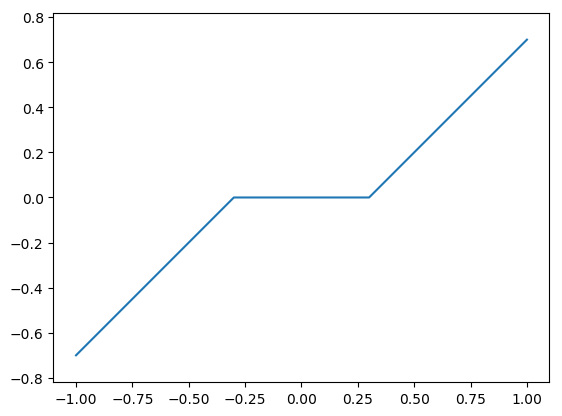Out:
(-1.1,1.1,-0.7699999999999999,0.7699999999999999)

The proximity operator of $\gamma$ times the indicator function of $\Omega$ is projection onto $\Omega$ and does not depends on $\gamma$. $$\mathrm{prox}_{\gamma f}(x)=\mathrm{prox}_{\iota_\Omega}(x)=P_\Omega(x) = x + A^* (A A^*)^{-1} (y-Ax).$$

In :
pA = pinv(A) # pseudo-inverse. Equivalent to pA = A.T.dot(inv(A.dot(A.T)))
prox_f = (x, y) -> x + pA*(y - A*x);


We set the values of $\gamma$ and $\rho$. Try different values to speed up the convergence.

In :
gamma = 0.1 # try 1, 10, 0.1
rho = 1     # try 1, 1.5, 1.9

Out:
1

Number of iterations.

In :
nbiter = 700;


### __Exercise: Implement nbiter iterations of the Douglas-Rachford algorithm. Keep track of the evolution of the $\ell^1$ norm.__

In :
s = zeros(N)
En_array = zeros(nbiter)
x = prox_f(s,y)
s += rho.*(prox_gamma_g(2.*x - s, gamma) - x)
En_array = maximum(sum(abs(x), 1))
for iter in 2 : nbiter  # iter goes from 1 to nbiter
x = prox_f(s,y)
s += rho.*(prox_gamma_g(2.*x - s, gamma) - x)
En_array[iter] = maximum(sum(abs(x), 1))
end
x_restored = x;


We display the original and the recovered signals.

In :
fig, (subfig1, subfig2) = subplots(1, 2, figsize = (16, 7)) # one figure with two horizontal subfigures
subfig1[:stem](xsharp)
subfig1[:set_ylim](0, 1.1)
subfig2[:stem](x_restored)
subfig2[:set_ylim](0, 1.1)
subfig1[:set_title](L"$x^\sharp$")
subfig2[:set_title](L"$x_\mathrm{restored}$")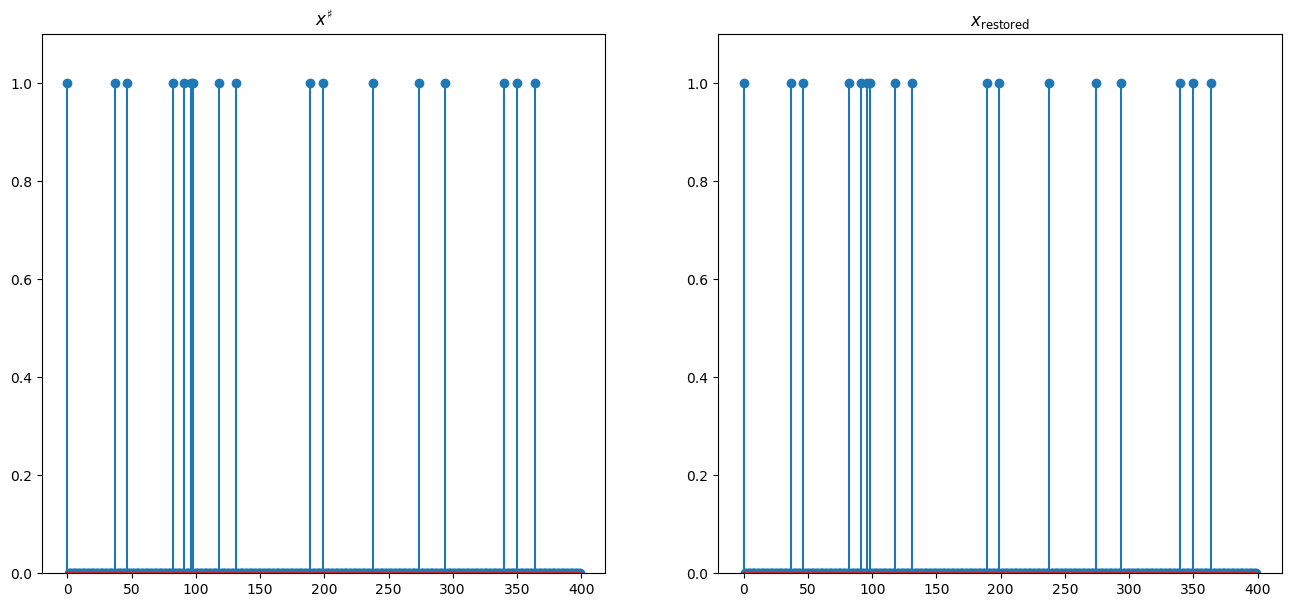Out:
PyObject <matplotlib.text.Text object at 0x00000000259878D0>

Since the original signal is highly sparse, it is perfectly recovered.

We display the convergence speed of the $\ell^1$ norm on the first half iterations, in log scale.

In :
plot(log10(En_array - minimum(En_array)))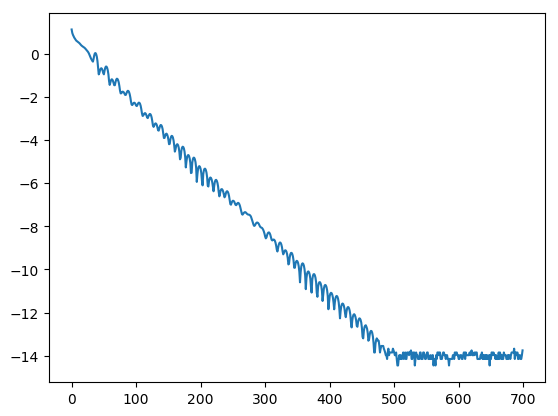Out:
1-element Array{Any,1}:
PyObject <matplotlib.lines.Line2D object at 0x0000000027467E48>

The convergence is linear in practice. Convergence up to machine precision (1e-14 here) is achieved after a few hundreds of iterations.

### __Exercise: test the recovery of a less sparse signal. What do you observe?__

In :
S = 31
srand(0)
sel = randperm(N)
sel = sel[1 : S]   # indices of the nonzero elements of xsharp
xsharp = zeros(N)
xsharp[sel] = 1

y = A*xsharp

gamma = 1
rho = 1
nbiter = 1000
s = zeros(N)
En_array = zeros(nbiter)
x = prox_f(s,y)
s += rho.*(prox_gamma_g(2*x - s, gamma) - x)
En_array = norm(x, 1)
for iter in 2 : nbiter
x = prox_f(s,y)
s += rho.*(prox_gamma_g(2*x - s, gamma) - x)
En_array[iter] = norm(x, 1)
end
x_restored = x

Out:
400-element Array{Float64,1}:
-1.08247e-15
1.0
-8.88178e-16
1.22125e-15
3.33067e-16
6.66134e-16
0.0
-1.88738e-15
3.16414e-15
-2.22045e-16
1.66533e-15
1.0
2.10942e-15
⋮
1.0
1.16573e-15
3.60822e-16
2.33147e-15
-3.33067e-16
-1.58207e-15
1.22125e-15
1.47105e-15
3.33067e-16
1.11022e-16
1.19349e-15
7.63278e-16
In :
fig, (subfig1, subfig2) = subplots(1, 2, figsize = (16, 7)) # one figure with two horizontal subfigures
subfig1[:stem](xsharp)
subfig1[:set_ylim](0, 1.1)
subfig2[:stem](x_restored)
subfig1[:set_title](L"$x^\sharp$")
subfig2[:set_title](L"$x_\mathrm{restored}$")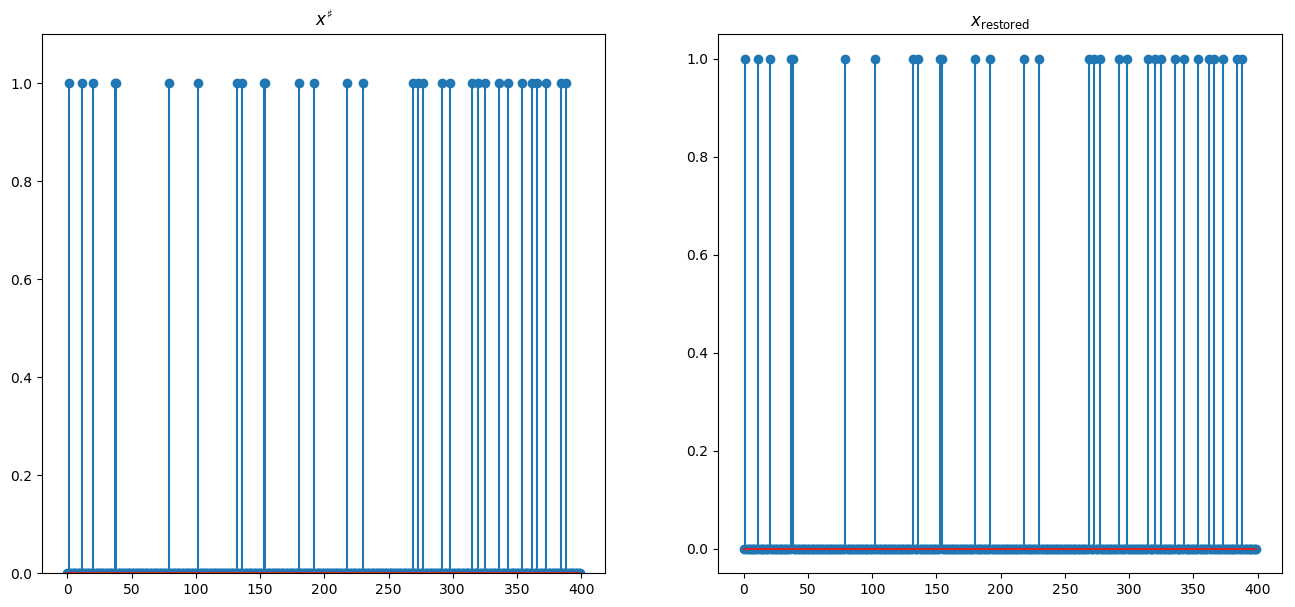Out:
PyObject <matplotlib.text.Text object at 0x000000002C70DFD0>
In :
plot(log10(En_array - minimum(En_array)))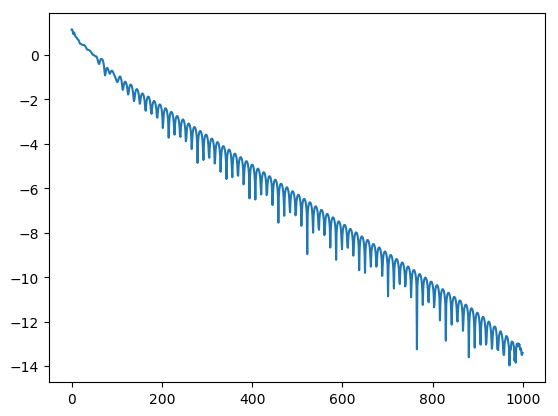Out:
1-element Array{Any,1}:
PyObject <matplotlib.lines.Line2D object at 0x000000002D64D240>

Convergence is much slower in this setting.